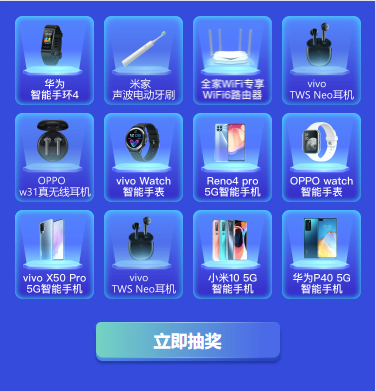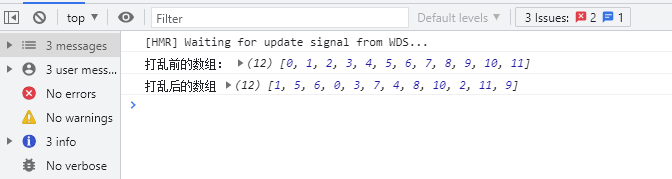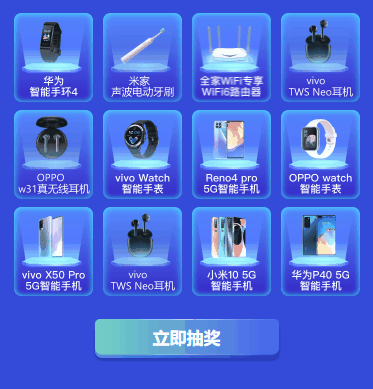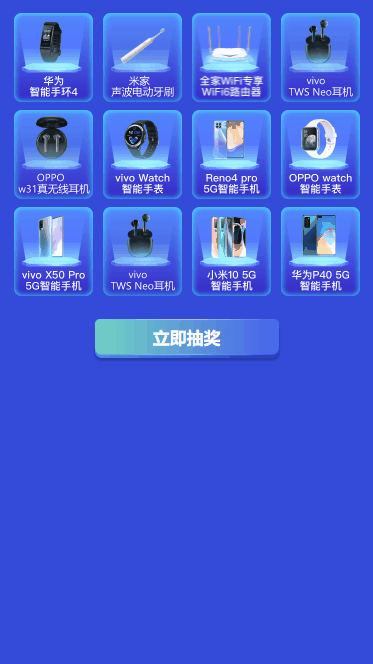# 1.需求1. 用户点击"立即抽奖"后12个奖品随机高亮
2. 请求接口，待所有产品都高亮后，根据接口结果，固定在抽中奖品上
3. 最后弹出中奖结果弹框

# 3.实现过程

## 3.1 奖品数据

prizes: [
{active: false, prizeName: '华为智能手环4', imgName: '1-huawei'},
{active: false, prizeName: '米家声波电动牙刷T300', imgName: '1-mijia'},
{active: false, prizeName: 'AX1800千兆双频路由器', imgName: '1-router'},
{active: false, prizeName: 'vivo TWS Neo耳机', imgName: '1-100m'},
{active: false, prizeName: 'OPPO w31真无线耳机', imgName: '1-oppo_w31'},
{active: false, prizeName: 'vivo Watch手表', imgName: '1-200m'},
{active: false, prizeName: 'reno4智能手机', imgName: '1-10y'},
{active: false, prizeName: '华为智能手表', imgName: '1-1g'},
{active: false, prizeName: 'vivo X5 Pro', imgName: '1-20g'},
{active: false, prizeName: 'vivo TWS Neo耳机', imgName: '1-vivo_neo'},
{active: false, prizeName: '小米10智能手机', imgName: '1-100y'},
{active: false, prizeName: 'vivo X50 Pro', imgName: '1-2g'}
]


## 3.2 高亮## 3.3 随机数组

//随机排列数，洗牌算法
shuffle(arr) {
let i = arr.length, t, j
while (i) {
j = Math.floor(Math.random() * (i--))
t = arr[i]
arr[i] = arr[j]
arr[j] = t
}
}


let arr = Array(this.prizes.length).fill(0).map((i, index) => index)
console.log("打乱前的数组：", arr)
//随机打乱数组元素
this.shuffle(arr)
console.log("打乱后的数组", arr)## 3.4 定时器&异步事件

let arr = Array(this.prizes.length).fill(0).map((i, index) => index)
let timeoutID = null
//模拟定时器
let sleep = time => new Promise((resolve) => {
timeoutID = setTimeout(resolve, time)
})
//定时任务函数，async/await
let collectEvent = async(count, interval) => {
for (let i = 0; i < count; i++) {
//等待上一个任务完成
await sleep(interval)
//按照随机数显示高亮
this.prizes.forEach((p, index) => { p.active = index === arr[i]  })
}
}
//产生随机数组
this.shuffle(arr)
//加入定时任务
collectEvent(arr.length, 300).then(() => {
clearTimeout(timeoutID)
})## 3.5 时间间隔

await sleep(interval)


await sleep(interval * i)


collectEvent(arr.length, 300)


collectEvent(arr.length, 100)## 3.6 中奖弹框

//生成随机奖品
getRandomIntInclusive(min, max) {
min = Math.ceil(min)
max = Math.floor(max)
return Math.floor(Math.random() * (max - min + 1)) + min
}


//加入定时任务
collectEvent(arr.length, 100).then(() => {
clearTimeout(timeoutID)
let checkedPrizeIndex = this.getRandomIntInclusive(0, this.prizes.length - 1)
this.prizes.forEach((p, index) => { p.active = index === checkedPrizeIndex })
this.prizeInfo = this.prizes[checkedPrizeIndex]
this.raffleResultShow = true
})# 4.总结posted @ 2021-08-17 17:06  nd  阅读(368)  评论(0编辑  收藏  举报